• 使用低通过滤器过滤图像
• 使用中值过滤器过滤图像
• 应用方向过滤器检测边缘
• 计算图像的拉普拉斯算子

# 使用低通过滤器过滤图像

## 操作步骤

cv::blur函数的目的是通过将每个像素替换为在矩形邻域上计算的平均像素值来使图像平滑。 该低通过滤器的应用如下：

   cv::blur(image,result,cv::Size(5,5));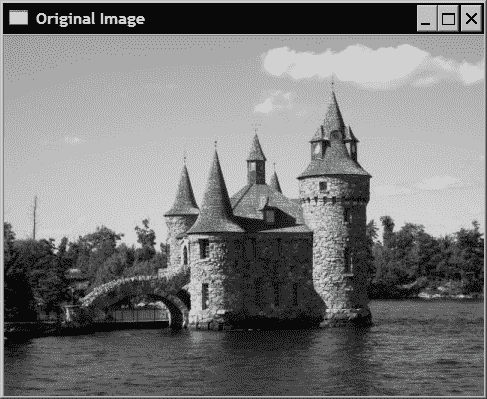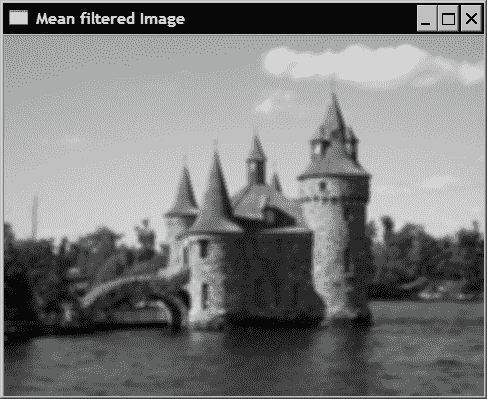cv::GaussianBlur(image,result,cv::Size(5,5),1.5);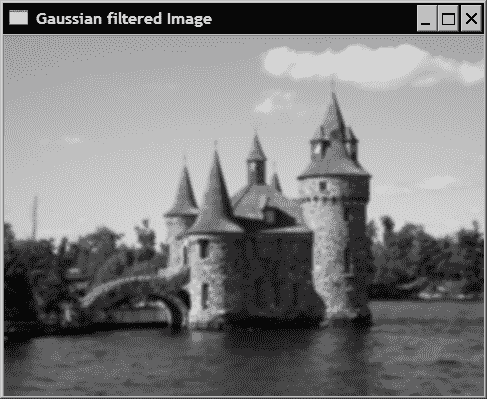## 工作原理

1/9 1/9 1/9
1/9 1/9 1/9
1/9 1/9 1/9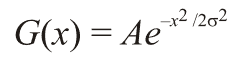[0.0 0.0 0.00026 0.10645 0.78657 0.10645 0.00026 0.0 0.0]


[0.00761 0.036075 0.10959 0.21345 0.26666
0.21345 0.10959 0.03608 0.00761 ]


cv::Mat gauss= cv::getGaussianKernel(9,sigma,CV_32F);


## 更多

cv::Mat reducedImage;  // to contain reduced image
cv::pyrDown(image,reducedImage); // reduce image size by half


cv::Mat resizedImage;  // to contain resized image
cv::resize(image,resizedImage,
cv::Size(image.cols/3,image.rows/3)); // 1/3 resizing


# 使用中值过滤器过滤图像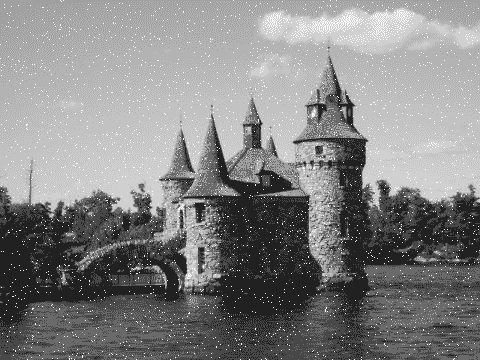## 操作步骤

   cv::medianBlur(image,result,5);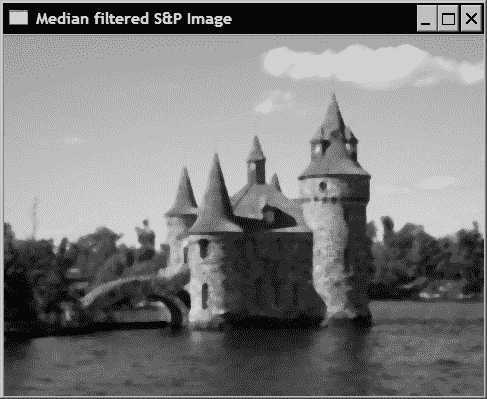## 工作原理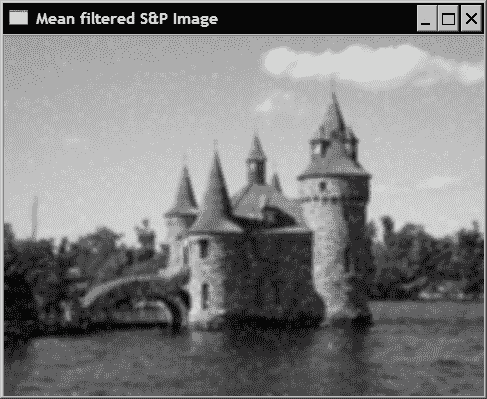# 应用方向过滤器检测边缘

## 操作步骤

   cv::Sobel(image,sobelX,CV_8U,1,0,3,0.4,128);


   cv::Sobel(image,sobelY,CV_8U,0,1,3,0.4,128);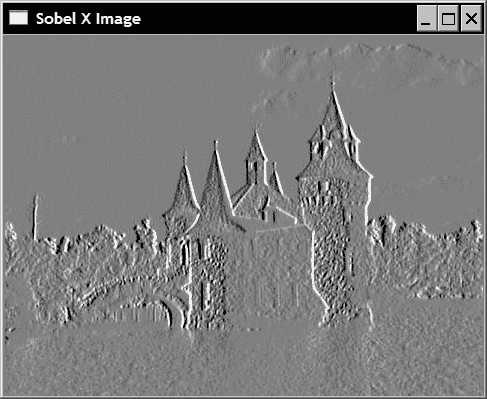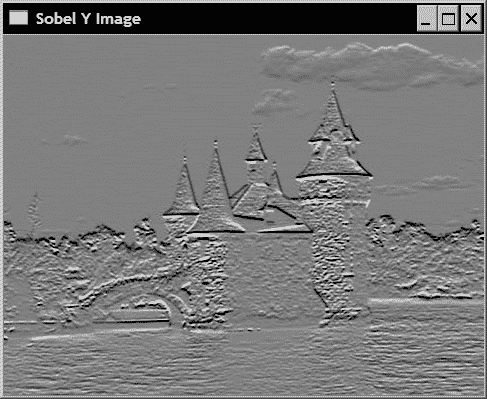// Compute norm of Sobel
cv::Sobel(image,sobelX,CV_16S,1,0);
cv::Sobel(image,sobelY,CV_16S,0,1);
cv::Mat sobel;
//compute the L1 norm
sobel= abs(sobelX)+abs(sobelY);


   // Find Sobel max value
double sobmin, sobmax;
cv::minMaxLoc(sobel,&sobmin,&sobmax);
// Conversion to 8-bit image
// sobelImage = -alpha*sobel + 255
cv::Mat sobelImage;
sobel.convertTo(sobelImage,CV_8U,-255./sobmax,255);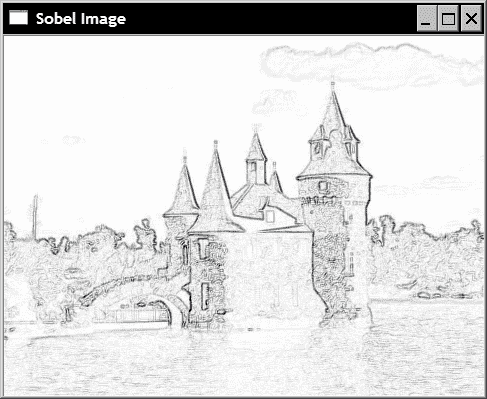cv::threshold(sobelImage, sobelThresholded,
threshold, 255, cv::THRESH_BINARY);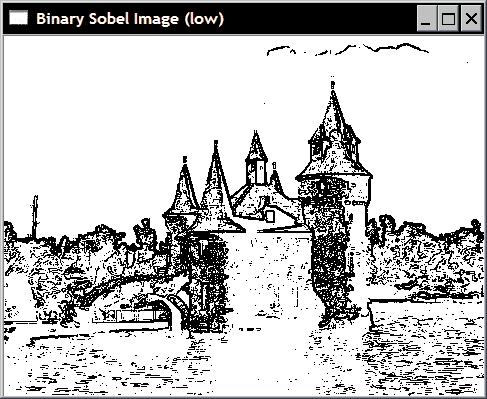## 工作原理

Sobel 运算符是经典的边缘检测线性过滤器，它基于简单的3x3核，其结构如下：

-1 0 1
-2 0 2
-1 0 1
-1 -2 -1
0 0 0
1 2 1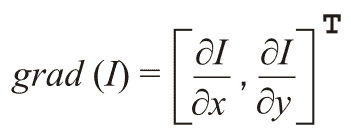cv::Sobel(image,  // input
sobel,  // output
image_depth,   // image type
xorder,yorder, // kernel specification
kernel_size,   // size of the square kernel
alpha, beta);  // scale and offset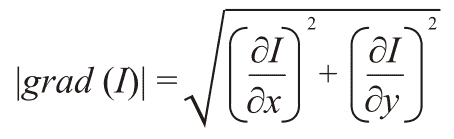//compute the L1 norm
sobel= abs(sobelX)+abs(sobelY);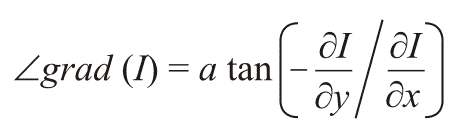// Sobel must be computed in floating points
cv::Sobel(image,sobelX,CV_32F,1,0);
cv::Sobel(image,sobelY,CV_32F,0,1);
// Compute the L2 norm and direction of the gradient
cv::Mat norm, dir;
cv::cartToPolar(sobelX,sobelY,norm,dir);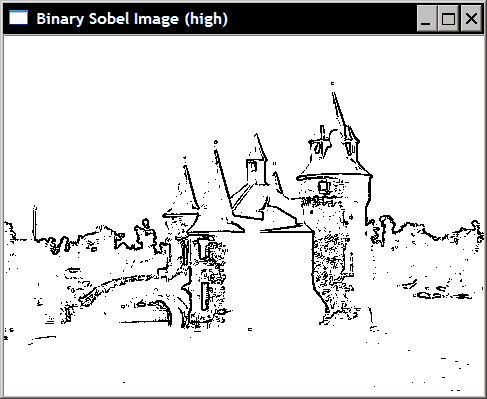## 更多

-1 0 1
-1 0 1
-1 0 1
-1 -1 -1
0 0 0
1 1 1

Roberts 运算符基于以下简单的2x2核：

1 0
0 -1
0 1
-1 0

-3 0 3
-10 0 10
-3 0 3
-3 -10 -3
0 0 0
3 10 3

   cv::Sobel(image,sobelX,CV_16S,1,0, CV_SCHARR);


   cv::Scharr(image,scharrX,CV_16S,1,0,3);


# 计算图像的拉普拉斯算子

## 操作步骤

OpenCV 函数cv::Laplacian计算图像的拉普拉斯算子。 它与cv::Sobel函数非常相似。 实际上，它使用相同的基本函数cv::getDerivKernels以获得其核矩阵。 唯一的区别是没有导数阶参数，因为根据定义，这些参数是二阶导数。

class LaplacianZC {

private:

// original image
cv::Mat img;

// 32-bit float image containing the Laplacian
cv::Mat laplace;
// Aperture size of the laplacian kernel
int aperture;

public:

LaplacianZC() : aperture(3) {}

// Set the aperture size of the kernel
void setAperture(int a) {

aperture= a;
}

// Compute the floating point Laplacian
cv::Mat computeLaplacian(const cv::Mat& image) {

// Compute Laplacian
cv::Laplacian(image,laplace,CV_32F,aperture);

// Keep local copy of the image
// (used for zero-crossings)
img= image.clone();

return laplace;
}


     // Get the Laplacian result in 8-bit image
// zero corresponds to gray level 128
// if no scale is provided, then the max value will be
// scaled to intensity 255
// You must call computeLaplacian before calling this
cv::Mat getLaplacianImage(double scale=-1.0) {

if (scale<0) {

double lapmin, lapmax;
cv::minMaxLoc(laplace,&lapmin,&lapmax);

scale= 127/ std::max(-lapmin,lapmax);
}

cv::Mat laplaceImage;
laplace.convertTo(laplaceImage,CV_8U,scale,128);

return laplaceImage;
}


   // Compute Laplacian using LaplacianZC class
LaplacianZC laplacian;
laplacian.setAperture(7);
cv::Mat flap= laplacian.computeLaplacian(image);
laplace= laplacian.getLaplacianImage();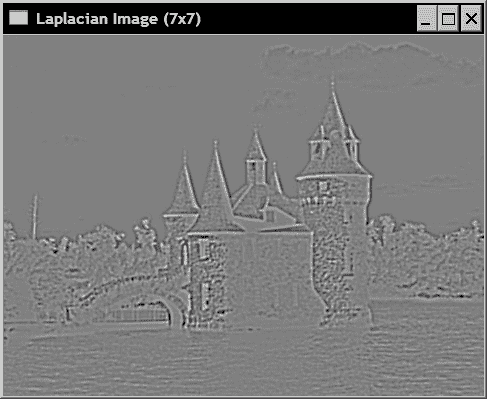## 工作原理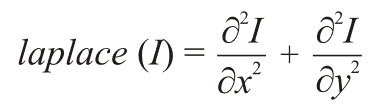0 1 0
1 -4 1
0 1 0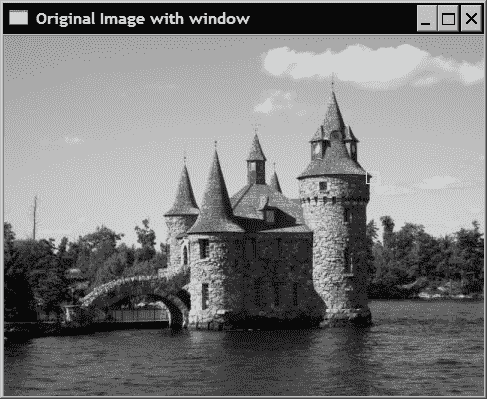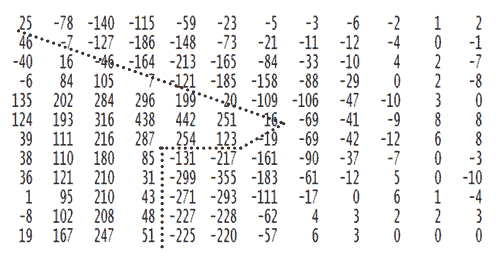// Get a binary image of the zero-crossings
// if the product of the two adjascent pixels is
// less than threshold then this zero-crossing
// will be ignored
cv::Mat getZeroCrossings(float threshold=1.0) {

// Create the iterators
cv::Mat_<float>::const_iterator it=
laplace.begin<float>()+laplace.step1();
cv::Mat_<float>::const_iterator itend=
laplace.end<float>();
cv::Mat_<float>::const_iterator itup=
laplace.begin<float>();

// Binary image initialize to white
cv::Mat binary(laplace.size(),CV_8U,cv::Scalar(255));
cv::Mat_<uchar>::iterator itout=
binary.begin<uchar>()+binary.step1();

// negate the input threshold value
threshold *= -1.0;

for ( ; it!= itend; ++it, ++itup, ++itout) {

// if the product of two adjascent pixel is
// negative then there is a sign change
if (*it * *(it-1) < threshold)
*itout= 0; // horizontal zero-crossing
else if (*it * *itup < threshold)
*itout= 0; // vertical zero-crossing
}

return binary;
}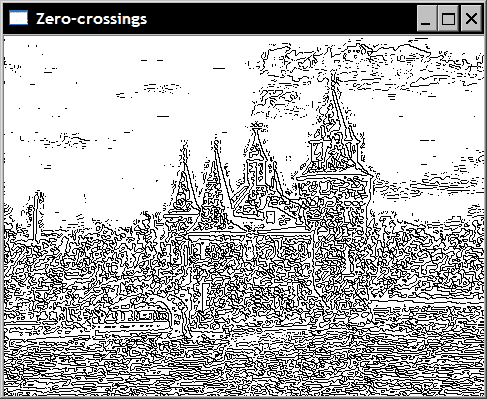0 -1 0
-1 5 -1
0 -1 0